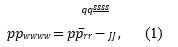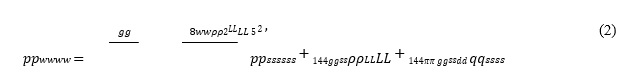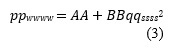# PE 7023 Advanced Production Design

Homework Assignment #1

Single-phase Liquid Inflow Performance Relationship (IPR) is given with the following equation:where, 𝑝𝑝̅𝑟𝑟 is the average reservoir pressure, 𝑝𝑝𝑤𝑤𝑤𝑤 is the bottom-hole flowing pressure, 𝑞𝑞𝑠𝑠𝑠𝑠 is the liquid flow rate at standard conditions, and 𝐽𝐽 is the productivity index.

Single-phase Liquid Outflow Performance Relationship (OPR) is given below for vertical upward flow assuming an incompressible liquid and constant friction factor, 𝑓𝑓.where, 𝑝𝑝𝑠𝑠𝑠𝑠𝑠𝑠 is the separator pressure, 𝑝𝑝𝑤𝑤𝑤𝑤 is the bottom-hole flowing pressure, 𝑞𝑞𝑠𝑠𝑠𝑠 is the liquid flow rate at standard conditions, 𝑔𝑔 is the acceleration gravity of Earth, 𝑔𝑔𝑠𝑠 is the unit conversion factor, 𝐿𝐿 is the depth of the well, 𝜌𝜌𝐿𝐿 is the density of the liquid and 𝑑𝑑 is the diameter of the tubing. Grouping the constant parameters, Eq. 2 can be written asQuestion:

1. Perform nodal analysis and find the solution point using the following input data:
 𝑝𝑝𝑠𝑠𝑠𝑠𝑠𝑠 100 psig 𝑝𝑝𝑟𝑟 5000 psig 𝐽𝐽 5 bbl/D/psi 𝐴𝐴𝐴𝐴𝐴𝐴 25 o 𝐿𝐿 8000 ft 𝑓𝑓𝑀𝑀𝑀𝑀𝑀𝑀𝑑𝑑𝑀𝑀 0.02 - 𝑇𝑇𝑇𝑇𝑇𝑇𝑇𝑇𝑇𝑇𝑔𝑔 𝐴𝐴𝐼𝐼 2.5 in
1. Plot nodal analysis plot taking bottom hole as the node
2. Plot nodal analysis plot taking wellhead as the node (Hint: rewrite Equations (1) and (2) for wellhead pressure 𝑝𝑝𝑤𝑤ℎ)
2. Develop an analytical expression for the stability of the Nodal Analysis Equilibrium Solution applying new stability analysis.
3. Write a computer code using a programming language of your choice to determine if the solution is stable or unstable
4. Run your computer code to check for stability:
1. By varying J and keeping B constant (Arbitrarily define the range for J)
2. By varying B and keeping J constant (Arbitrarily define the range for B. You can change any parameter included in the definition of B to have a meaningful B range)
5. Present your stability results on a J vs. B (or variable of your choice included in the definition of B) map